## Year 2 maths worksheet: Multiplication in any order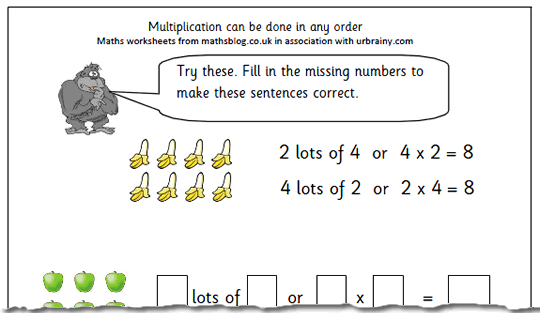Here we have a maths worksheet, one of a set from urbrainy.com, which looks at a vital part of understanding the process of multiplication: that it can be done in any order. This is also true of addition, but not of subtraction or division. Continue reading “Year 2 maths worksheet: Multiplication in any order”

## Mangahigh Review: Online maths for High School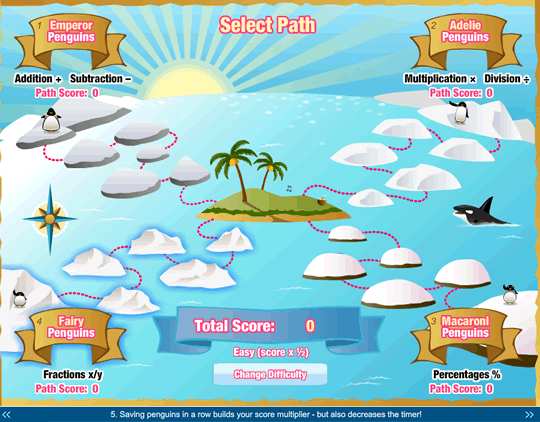A relatively new site which has come to my attention recently is mangahigh.com. Aimed at High School students, this would only be appropriate to older primary children with a good mathematical knowledge.

A great free game to try out would be Ice Ice Maybe on estimation which is well within the range of older primary children – but watch out, it might prove addictive!

The aim of the site is to intrigue and interest students with high quality games whilst at the same time introducing new maths concepts. The repetition within playing the game leads to knowledge and success in exams.

As they say:
“Mangahigh’s unique game-based learning system brings out the mathematician in ALL students who love games.”

The games are certainly of a high standard, equal to many of the best on-line games and will almost certainly be attractive to High School students.

The games award students for success but more than this, it has a ‘learning engine’ that includes thousands of maths problems with worked answers.

Mangahigh covers all of the lessons required to score highly at Foundation and Higher Maths GCSE exams, and the questions and content are matched to teaching goals for all the major UK exam boards.

The people behind mangahigh certainly give it credibility: Ian Livingstone of Livingstone and Jackson Dungeons and Dragons and White Dwarf magazine as well as Marcus Du Sautoy, Professor of Maths at Oxford and Toby Rowland of King.com.

Starting at £4.85 a month if subscribing for a year there are many worse ways of spending your money on your children.

## Coming Soon: Multiplication and percentagesTwo maths worksheets for year 2 coming up next week. One looks at a vital part of understanding the process of multiplication: that it can be done in any order. This is also true of addition, but not of subtraction or division.
Setting up a little shop and buying and selling things has always been a great part of early maths, but usually this activity is limited to adding up and giving change. It can be used equally well to reinforce multiplication skills, as our worksheet shows.
We will also be publishing the second of our percentages worksheets for Year 5. It is typical of the English that we manage to write per cent in two different ways. Unlike the Americans who use ‘percent’, we use two words ‘per cent’, apart from when we write ‘percentage’ when we put it altogether.

## Maths worksheet: Year 5 negative numbers (2)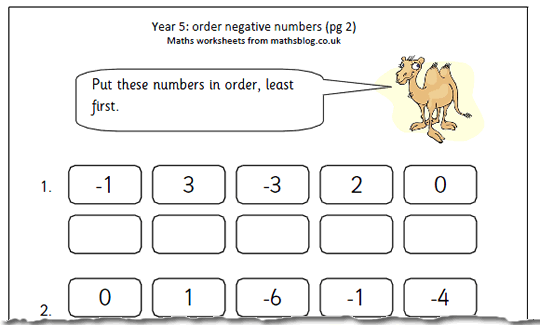Here is the second of our pages on negative numbers, involving ordering 5 numbers from the least to the most.

This is probably a good time to distinguish between using the ‘minus’ sign to perform a subtraction and using it to indicate a negative number. In my time at school the word ‘minus’ was used for both and we learnt all sorts of tricks such as ‘two minuses make a plus’ which are not always as helpful to understanding as at first thought. Continue reading “Maths worksheet: Year 5 negative numbers (2)”

## Resource of the Week: Venn diagrams and triangles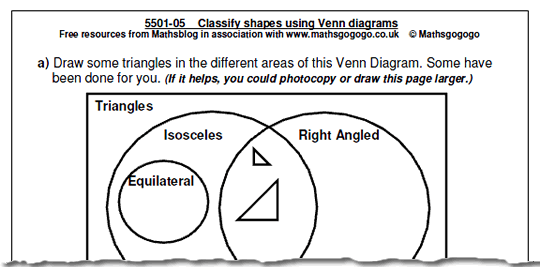Most of the maths worksheets you find free on the internet tend to be number based. But not here on mathsblog! There are two parts to this free maths worksheet on classifying shapes, both involving Venn diagrams. The idea is to put either the names of shapes or drawings of shapes in the correct areas of the Venn diagram. Continue reading “Resource of the Week: Venn diagrams and triangles”

## Maths Worksheet: Divide by 100 mentally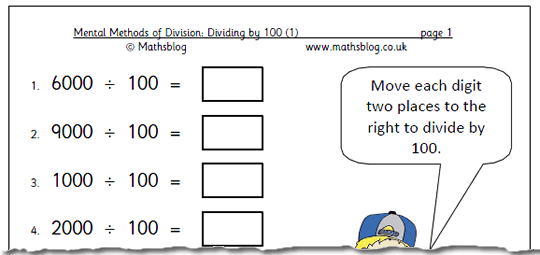Here we have the next worksheet in our series of dividing mentally. This page looks at dividing larger numbers by 100. All the numbers are multiples of 100 so there will be no decimals involved with the answers. As has been said before, but the idea has to be repeated many times for children to fully understand; to divide by 10, move each digit one place to the right. To divide by 100 move each digit two places to the right. Continue reading “Maths Worksheet: Divide by 100 mentally”

## Maths worksheet: multiplication as repeated addition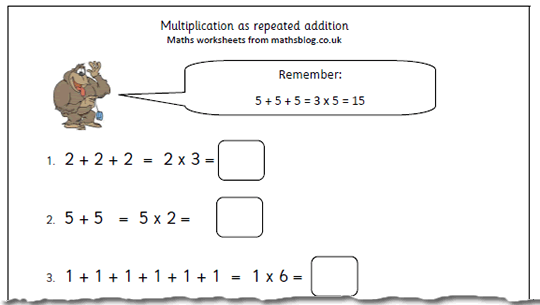One of the best ways of explaining multiplication is to think of it as repeated addition. So, a multiplication such as 4 x 3 is the same as adding 4 three times (4 + 4 + 4) or 3 lots of 4. Of course, 3 lots of 4 is the same as 4 lots of 3 which implies that multiplication can be done in any order, unlike division or subtraction. Continue reading “Maths worksheet: multiplication as repeated addition”

## Coming soon: Multiplication, division and negative numbersOne of the best ways of explaining multiplication is to think of it as repeated addition. So, a multiplication such as 4 x 3 is the same as adding 4 three times (4 + 4 + 4) or 3 lots of 4. We have an excellent page on this coming up next week, thanks to urbrainy.com.

We also have the next worksheet in our series of dividing mentally. This page will look at dividing larger numbers by 100. All the numbers are multiples of 100 so there will be no decimals involved with the answers.

Ordering negative numbers can prove tricky but we have a great page on this next week, suitable for Year 5 children. Putting two numbers in order on a number line follows one simple rule; the number to the right is always the larger number of the two. So -3 is smaller than -1.

## Maths worksheet: Converting metric units (2)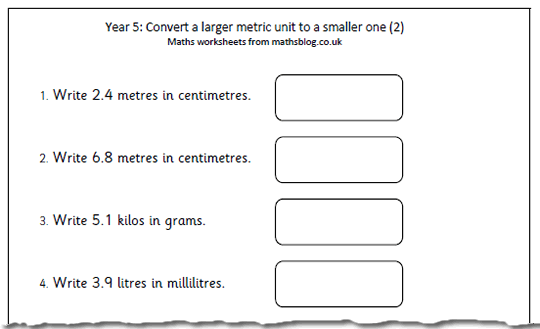This is another maths worksheet which looks at converting a larger metric unit to a smaller one, and is suitable for Year 5 children who have a good grasp of the metric system. Even so, don’t be surprised if children make mistakes with these, especially when multiplying by ten, one hundred or one thousand. Continue reading “Maths worksheet: Converting metric units (2)”

## Resource of the Week: Counting in twos

If you have young children don’t forget to go to our fun maths games. Here we have Colin Caterpillar and his chums who love eating apples two at a time. Help him count up in twos. There are plenty more in our Free Maths Interactive Games section aimed at helping 5/6 year old children with counting, addition, subtraction etc.